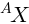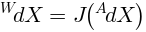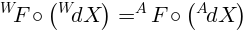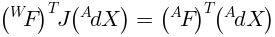## How static forces transform between kinematic frames

The lever princple is a clear way to see how displacements and forces are related in a simple one-joint kinematic system. Visualize a seesaw with the pivot offset from the middle. To balance a pair of objects, each must have a distance from the pivot inversely proportional to its weight.

This idea can be generalized to handle transformations between any pair of kinematic coordinate systems. The usual situation is transformation between actuator coordinates - the actuator displacements and/or rotations - and world coordinates, the usual x,y,z,roll,pitch,yaw.

We only need to know the differential relationship between displacements in each system to find the transformation rule for forces. The key idea is the priciple of virtual work: The total energy expended in an infinitesimal displacement of one system must be same in any other (kinematically connected) system. To make life easier, we only need to know the displacement transformation in the one direction. We are free to choose the easy way, which usually turns out to be world-to-joint.

The dot product of two vectors expresssed in matrix notation is:We use prefix superscripts to denote frames. A point X in the world frame is:The same point X in the actuator frame A is:Frames are related by transformations. A transformation T from actuator to world is:The Jacobian matrix of a frame transformation relates infinitesimal displacements:The total work done by a force F acting over an infinitesimal displacement is the same in either frame:Expressed in matrix form this becomes:Using the definition of the Jacobian:Removing the common right operand from both sides, we have the operator formula:There is a matrix identity:Using this identity on the operator formula and transposing both sides we obtain:So the world force is given by:Which we can compare with the infinitesimal displacement transform:Comparing the last two expressions confirms the seesaw analogy: If the expressions were scalars and J was the lever arm ratio, we have exactly the expected relationship.

Quod Erat Demonstrandum

The equations on this page were typeset for web publication using JEuler.